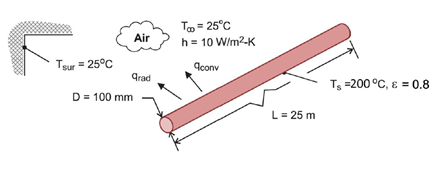## A Steam Pipe

Consider an overhead 25-m long, 100-mm diameter, uninsulated steam pipe is routed through a building whose surrounding walls and air are at 25°C, while pressurized steam maintains a pipe surface temp of 200°C. With the natural convection coefficient of h = 10 W/m^2(K), and surface emissivity of 0.8, what is the rate of heat loss from the steam line?Hint
In this scenario, both convection and radiation are simultaneously occurring:
$$q=q_{conv}+q_{rad}$$$Hint 2 For radiation emitted by a body: $$q=\varepsilon \sigma AT^4$$$
where $$\varepsilon$$ is the body’s emissivity, $$\sigma$$ is the Stefan-Boltzmann constant $$=5.67 \times 10^{-8}W/(m^2 \cdot K^4)$$ , $$A$$ is the body’s surface area, and $$T$$ is the absolute temperature.
ASSUMPTIONS : (1) The steam line continuously operates throughout the year, (2) Net radiation transfer is between a small surface (steam line) and a large enclosure (plant walls).
ANALYSIS : In this scenario, convection and radiation are both simultaneously occurring. Thus, the heat loss can be expressed as:
$$q=q_{conv}+q_{rad}$$$For radiation emitted by a body: $$q=\varepsilon \sigma AT^4$$$
where $$\varepsilon$$ is the body’s emissivity, $$\sigma$$ is the Stefan-Boltzmann constant $$=5.67 \times 10^{-8}W/(m^2 \cdot K^4)$$ , $$A$$ is the body’s surface area, and $$T$$ is the absolute temperature.
For convection, which is the heat transfer from a heat source to a cooler object via the mass motion of a fluid, Newton’s Law of Cooling applies:
$$q=h A(T_w-T_\infty )$$$where $$h$$ is the fluid’s convection heat-transfer coefficient, $$A$$ is the convection surface area, $$T_w$$ is the wall surface temperature, and $$T_\infty$$ is the bulk fluid temperature. Combining both modes of heat transfer into a single equation: $$q=q_{conv}+q_{rad}=A[h(T_{s}-T_\infty )+\varepsilon \sigma (T_{s}^4-T_{sur}^4)]$$$
where
$$A=\pi DL=\pi (0.1m)(25m)=7.85m^2$$$Finally: $$q=7.85m^2[10\frac{W}{m^2K}(200-25)K+0.8\cdot 5.67\times10^{-8}\frac{W}{m^2K^4}((273+200)^4-(273+25)^4)K^4]$$$
$$q=7.85m^2[1750\frac{W}{m^2}+1912.76\frac{W}{m^2}]=28,753\:W$$\$
28,753 W